# 庆祝Lazarus1.0发布！Delphi和Freepascal的性能比较

### 时间：2012/9/6 10:49:22 点击：核心提示：好久没有关注freepascal和lazarus，前两天一看，lazarus居然已经发布了1.0正式版，下载了一个windows版，一试，感觉比较爽，IDE好像已经强过DELPHI7（我一直用DELPHI7），中文的问题好像已经没有了。有关生成的可执行的文件太大的问题也试了一下，缺省的文件还是比较大...

（1）平衡二叉树的创建

const
cBase64 = 'ABCDEFGHIJKLMNOPQRSTUVWXYZabcdefghijklmnopqrstuvwxyz0123456789+/=';
cBase64Len=Length(cBase64);

procedure TForm1.Button1Click(Sender: TObject);
var
i:Integer;
Node:TAVLTreeNode;
Image:TImage;
T1,T2:TDateTime;
begin
T1:=Now;

Tree.Clear;

RandSeed:=1;

DrawCount:=1;

for i:=1 to 20*10000 do
begin
DrawCount:=(DrawCount+1) mod 2;
Image:=TImage(FindComponent('Image'+IntToStr(DrawCount+1)));
//  Image.Canvas.FillRect(Image.ClientRect);
//  Tree.DrawTreeNode(Image.Canvas);

Node:=TAVLTreeNode.Create;
Node.Key:=cBase64[Random(cBase64Len)+1];
//  ShowMessage('Button1: Begin'+IntToStr(i)+' '+Node.Key);
DrawCount:=(DrawCount+1) mod 2;

Image:=TImage(FindComponent('Image'+IntToStr(DrawCount+1)));
//  Image.Canvas.FillRect(Image.ClientRect);
//  Tree.DrawTreeNode(Image.Canvas);

//  ShowMessage('Button1: End'+IntToStr(i));
end;
T2:=Now;
ShowMessage(IntToStr(Round((t2-t1)*24*3600*1000)));
end;

DELPHI耗时234ms
Freepascal耗时141ms

（2）平衡二叉树的释放和创建

DELPHI耗时328ms
Freepascal耗时187ms

（3）平衡二叉树的查找
var
s:string;
i:Integer;
T1,T2:TDateTime;
begin
T1:=Now;
for i:=1 to 1000*1000 do
begin
s:=cBase64[Random(cBase64Len)+1];
Tree.Find(s);
end;
T2:=Now;
ShowMessage(IntToStr(Round((t2-t1)*24*3600*1000)));
end;

DELPHI耗时218ms
Freepascal耗时141ms

（4）数学运算
var
s:string;
i:Integer;
T1,T2:TDateTime;
begin
T1:=Now;
for i:=1 to 1000*1000 do
begin
RandSeed:=round(math.power(10.5,3.2)+log10(1000*25*123)+sqrt(22*55)+math.sinh(1.2)*100)*100;
//  s:=cBase64[Random(cBase64Len)+1];
//  Tree.Find(s);
end;
T2:=Now;
ShowMessage(IntToStr(Round((t2-t1)*24*3600*1000)));
end;

DELPHI耗时235ms
Freepascal耗时218ms

（5）在画布上显示
var
i:Integer;
Node:TAVLTreeNode;
Image:TImage;
T1,T2:TDateTime;
begin
T1:=Now;

Tree.Clear;

RandSeed:=1;

DrawCount:=1;

for i:=1 to 200 do
begin
DrawCount:=(DrawCount+1) mod 2;
Image:=TImage(FindComponent('Image'+IntToStr(DrawCount+1)));
Image.Canvas.FillRect(Image.ClientRect);
Tree.DrawTreeNode(Image.Canvas);

Node:=TAVLTreeNode.Create;
Node.Key:=cBase64[Random(cBase64Len)+1];
//  ShowMessage('Button1: Begin'+IntToStr(i)+' '+Node.Key);
DrawCount:=(DrawCount+1) mod 2;

Image:=TImage(FindComponent('Image'+IntToStr(DrawCount+1)));
Image.Canvas.FillRect(Image.ClientRect);
Tree.DrawTreeNode(Image.Canvas);

//  ShowMessage('Button1: End'+IntToStr(i));
end;
T2:=Now;
ShowMessage(IntToStr(Round((t2-t1)*24*3600*1000)));
end;

DELPHI耗时312ms
Lazarus耗时688ms

###### 共有评论 2 条相关评论###### 发表我的评论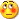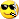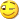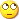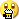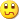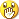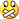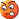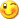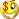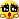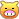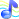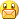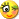• 大名：
• 内容：
• 没有
• 没有